• 决定系数MATLAB代码实现return_matlab 一组简单的函数来计算回归。 成对的输入值和目标值随机分布在训练，验证和测试集中。 使用训练集theta的值可以针对任何假设进行计算。 Lambda是通过检测成本函数的最佳值来确定...
• 该文件可用于直接计算数据集的决定系数 (R2)。 两个输入参数：'X' 和 'Y' 一个输出参数：'R2' X：x 参数的向量Y：y 参数的向量R2：决定系数 输入语法：rsquare(X,Y) 由 Joris Meurs BASc (2016) 开发matlab
• 计算数据拟合模型和RMSE的确定系数[r2 rmse] = rsquare(y,f) [r2 rmse] = rsquare(y,f,c) RSQUARE 计算确定系数（R 方）值实际数据Y和模型数据F。代码使用通用版本R-square，基于比较估计误差的可变性与原始值的可变...matlab
• 这个 m 文件计算预测的近似决定系数 (R2)，比如 R2pred = 1 - (PRESS/SST) 其中 PRESS = 预测误差平方和，SST = 总平方和。 该统计数据给出了回归模型预测能力的一些指示。 因此，与最小二乘拟合解释的原始数据中...matlab
• ## 决定系数

万次阅读 2016-09-17 10:42:41
在对数据进行线性回归计算之后,我们能够得出相应函数的系数, 那么我们如何知道得出的这...所以我们用到了一种方法叫 coefficient of determination (决定系数) 来判断 回归方程 拟合的程度. 首先我们先定义几个概念
概念引用：http://blog.csdn.net/ytdxyhz/article/details/51730995
在对数据进行线性回归计算之后,我们能够得出相应函数的系数, 那么我们如何知道得出的这个系数对方程结果的影响有强呢?
所以我们用到了一种方法叫 coefficient of determination (决定系数) 来判断 回归方程 拟合的程度.  首先我们先定义几个概念   1. Sum Of Squares Due To Error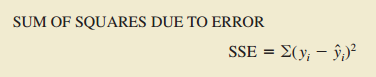对于第i个观察点, 真实数据的Yi与估算出来的Yi-head的之间的差称为第i个residual, SSE 就是所有观察点的residual的和 2. Total Sum Of Squares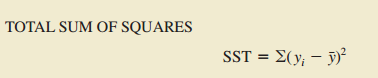3. Sum Of Squares Due To Regression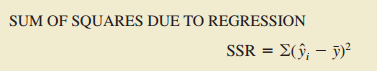通过以上我们能得到以下关于他们三者的关系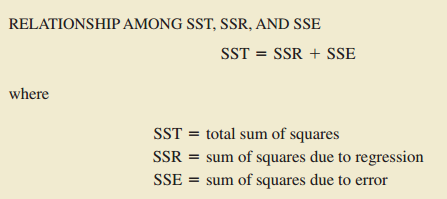决定系数: 判断 回归方程 的拟合程度   (coefficient of determination)决定系数也就是说: 通过回归方程得出的 dependent variable 有 number% 能被 independent variable 所解释. 判断拟合的程度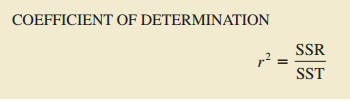(Correlation coefficient) 相关系数 : 测试dependent variable 和 independent variable 他们之间的线性关系有多强. 也就是说, independent variable 产生变化时 dependent variable 的变化有多大.

可以反映是正相关还是负相关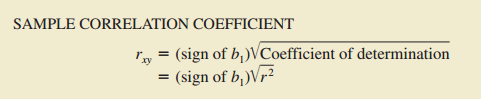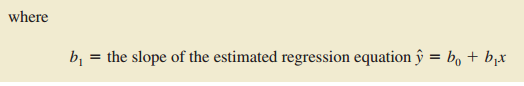Udacity：机器学习纳米工程师学位

如果不能对模型的训练和测试的表现进行量化地评估，我们就很难衡量模型的好坏。通常我们会定义一些衡量标准，这些标准可以通过对某些误差或者拟合程度的计算来得到。在这个项目中，你将通过运算决定系数R2 来量化模型的表现。模型的决定系数是回归分析中十分常用的统计信息，经常被当作衡量模型预测能力好坏的标准。
R2的数值范围从0至1，表示目标变量的预测值和实际值之间的相关程度平方的百分比。一个模型的R2 值为0说明它完全无法预测目标变量；而一个R2 值为1的模型则可以对目标变量进行完美的预测。从0至1之间的数值，则表示该模型中目标变量中有百分之多少能够用特征来解释。_模型也可能出现负值的R2，这种情况下模型所做预测还不如直接计算目标变量的平均值。
# TODO: Import 'r2_score'
from sklearn.metrics import r2_score

def performance_metric(y_true, y_predict):
""" Calculates and returns the performance score between
true and predicted values based on the metric chosen. """

# TODO: Calculate the performance score between 'y_true' and 'y_predict'
score = r2_score(y_true, y_predict)

# Return the score
return score

#print performance_metric()


展开全文机器学习 R2
• 确定系数matlab计算代码CODY1.0源代码 ======================================== CODY - 一个 MATLAB(R) 动态包肠道微生物群的建模 版本：1.0 主页：稍后添加 作者：耿军 Friday, Feb. 23, 2019 % 由 Jun Geng，...
• 文章目录相关系数（Correlation coefficient）决定系数（coefficient of determination） 相关系数（Correlation coefficient） 皮尔逊相关系数 也称为简单相关系数，用于研究变量之间 线性相关的程度。相关系数...


文章目录
相关系数（Correlation coefficient）决定系数（coefficient of determination）

相关系数（Correlation coefficient）
皮尔逊相关系数 也称为简单相关系数，用于研究变量之间 线性相关的程度。相关系数可以用简写

c

c

cc

表示，不过通常还是会用

r

r

来表示。
NOTE：皮尔逊相关系数并不是唯一的相关系数，但是最常见的相关系数。
定义：

ρ

x

y

=

r

(

X

,

Y

)

=

C

o

v

(

X

,

Y

)

V

a

r

[

X

]

V

a

r

[

Y

]

\rho_{xy} = r(X,Y) = \frac{Cov(X,Y)}{\sqrt{Var[X]Var[Y]}}

其中，

C

o

v

(

X

,

Y

)

Cov(X,Y)

是

X

X

和

Y

Y

的协方差，

V

a

r

[

X

]

Var[X]

和

V

a

r

[

Y

]

Var[Y]

分别为

X

X

，

Y

Y

的方差。
值域：[-1,1]
意义：定量刻画了

X

X

和

Y

Y

的相关程度，

∣

ρ

x

y

∣

|\rho_{xy}|

越大则相关程度越大；

∣

ρ

x

y

∣

=

0

|\rho_{xy}| = 0

对应相关程度最低。

ρ

x

y

\rho_{xy}

为 正数 时表示两个变量呈 正相关，即一个变量增大时另一个变量也增大（比如气温越高，冰淇淋的销量就越多）；

ρ

x

y

\rho_{xy}

结果为 负数 时两个变量呈 负相关，即一个变量增大时另一个变量减小（比如海拔越高时，空气中的氧气含量就越少）；

ρ

x

y

\rho_{xy}

为 0，则表示两个变量不为线性关系，有可能两者不相关，但也有可能两者有更加复杂的关系。
相关性的强弱大致可以按照如下分布来进行判定：

∣

ρ

x

y

∣

|\rho_{xy}|

0.8 ~ 1.0，极强相关

∣

ρ

x

y

∣

|\rho_{xy}|

0.6 ~ 0.8，强相关

∣

ρ

x

y

∣

|\rho_{xy}|

0.4 ~ 0.6，中等程度相关

∣

ρ

x

y

∣

|\rho_{xy}|

0.2 ~ 0.4，弱相关

∣

ρ

x

y

∣

|\rho_{xy}|

0.0 ~ 0.2，极弱相关或无相关
计算方法：
方法一：Excel 自带公式
公式 -> 插入函数 -> 统计 -> CORREL
或者直接在 Excel 表格任意空白位置输入：=CORREL()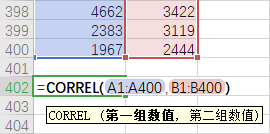方法二：专业数据分析工具 SPSS
参考文章： 皮尔逊积矩相关系数 【从零开始的AI学习】如何判断两个数据之间的相关性？

决定系数（coefficient of determination）
决定系数也称为拟合优度，是 相关系数的平方。用于 评价拟合的好坏，这里的拟合可以是线性或非线性的。通常记作

r

2

r^2

。
意义：决定系数

r

2

r^2

约接近于 1，则拟合回归的效果越好。
表示可根据自变量的变异来解释因变量的变异部分。如某学生在某智力量表上所得的 IQ 分与其学业成绩的相关系数 r=0.66，则决定系数 R^2=0.4356，即该生学业成绩约有 44%可由该智力量表所测的智力部分来说明或决定。
参考文章： 决定系数 统计-R(相关系数)与R^2(决定系数)傻傻分不清
展开全文• matlab决定因数代码使用“Fminsearch”（matlab）求解 Pitzer 方程 使用“Pitzer 方程”对磷酸进行热力学建模： 描述： 本研究中提出了一种计算方法来评估冰点和磷酸水溶液的等压摩尔浓度的 Pitzer 模型的离子相互...
• ## 决定系数R2

千次阅读 2019-03-19 16:33:03
决定系数 表示：y的波动有多少能被你和直线所描述。 当直线拟合的很好，y到拟合线的差值会很小，此时决定系数接近1.表示y值基本都被拟合直线所描述了。 ...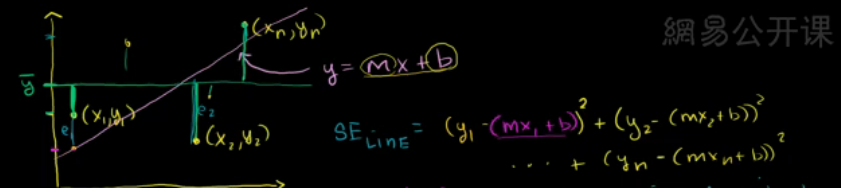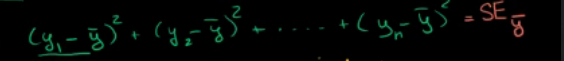决定系数 表示：y的波动有多少能被你和直线所描述。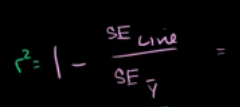当直线拟合的很好，y到拟合线的差值会很小，此时决定系数接近1.表示y值基本都被拟合直线所描述了。
展开全文• ## 相关系数r和决定系数R2的那些事

万次阅读 多人点赞 2019-01-07 11:09:28
文章目录相关系数$r$和决定系数$R^2$的那些事协方差与相关系数决定系数（R方）参考资料 相关系数rrr和决定系数R2R^2R2的那些事 有人说相关系数（correlation coefficient，rrr）和决定系数（coefficient of ...


文章目录
相关系数$r$和决定系数$R^2$的那些事协方差与相关系数决定系数（R方）参考资料

相关系数

r

r

和决定系数

R

2

R^2

的那些事
有人说相关系数（correlation coefficient，

r

r

）和决定系数（coefficient of determination，

R

2

R^2

，读作R-Squared）都是评价两个变量相关性的指标，且相关系数的平方就是决定系数？这种说法对不对呢？请听下文分解！
协方差与相关系数
要说相关系数，我们先来聊聊协方差。在之前的博文《使用Python计算方差协方差相关系数》中提到协方差是计算两个随机变量

X

X

和

Y

Y

之间的相关性的指标，定义如下：

C

o

v

(

X

,

Y

)

=

E

[

(

X

−

E

X

)

(

Y

−

E

Y

)

]

\mathrm{Cov}(X, Y) = \mathrm{E}[(X - \mathrm{E}X)(Y - \mathrm{E}Y)]

但是协方差有一个确定：它的值会随着变量量纲的变化而变化（covariance is not scale invariant），所以，这才提出了相关系数的概念：

r

=

C

o

r

r

(

X

,

Y

)

=

C

o

v

(

X

,

Y

)

σ

X

⋅

σ

Y

=

E

[

(

X

−

E

X

)

(

Y

−

E

Y

)

]

E

[

X

−

E

X

]

2

E

[

Y

−

E

Y

]

2

r = \mathrm{Corr}(X, Y) = \frac{Cov(X, Y)}{\sigma_X \cdot \sigma_Y} = \frac{\mathrm{E}[(X - \mathrm{E}X)(Y - \mathrm{E}Y)]}{\sqrt{\mathrm{E}[X - \mathrm{E}X]^2}\sqrt{\mathrm{E}[Y - \mathrm{E}Y]^2}}

对于相关系数，我们需要注意：
相关系数是用于描述两个变量线性相关程度的，如果

r

&gt;

0

r \gt 0

，呈正相关；如果

r

=

0

r = 0

，不相关；如果

r

&lt;

0

r \lt 0

，呈负相关。如果我们将

X

−

E

X

X - \mathrm{E}X

和

Y

−

E

Y

Y - \mathrm{E}Y

看成两个向量的话，那

r

r

刚好表示的是这两个向量夹角的余弦值，这也就解释了为什么

r

r

的值域是[-1, 1]。相关系数对变量的平移和缩放（线性变换）保持不变（Correlation is invariant to scaling and shift，不知道中文该如何准确表达，?）。比如

C

o

r

r

(

X

,

Y

)

=

C

o

r

r

(

a

X

+

b

,

Y

)

\mathrm{Corr}(X, Y) = \mathrm{Corr}(aX + b, Y)

恒成立。
决定系数（R方）
下面来说决定系数，R方一般用在回归模型用用于评估预测值和实际值的符合程度，R方的定义如下：

R

2

=

1

−

F

V

U

=

1

−

R

S

S

T

S

S

=

1

−

∑

i

(

y

i

−

f

i

)

2

∑

i

(

y

i

−

y

^

)

2

R^2 = 1 - \mathrm{FVU} = 1 - \frac{\mathrm{RSS}}{\mathrm{TSS}} = 1 - \frac{\sum\limits_i(y_i - f_i)^2}{\sum\limits_i(y_i - \hat{y})^2}

上式中

y

y

是实际值，

f

f

是预测值，

y

^

\hat{y}

是实际值的平均值。

F

V

U

\mathrm{FVU}

被称为fraction of variance unexplained，RSS叫做Residual sum of squares，TSS叫做Total sum of squares。根据

R

2

R^2

的定义，可以看到

R

2

R^2

是有可能小于0的，所以

R

2

R2

不是

r

r

的平方。一般地，

R

2

R^2

越接近1，表示回归分析中自变量对因变量的解释越好。
对于

R

2

R^2

可以通俗地理解为使用均值作为误差基准，看预测误差是否大于或者小于均值基准误差。
此外，我们做这样一个变形：

R

2

=

1

−

∑

i

(

y

i

−

f

i

)

2

/

n

∑

i

(

y

i

−

y

^

)

2

/

n

=

1

−

R

M

S

E

V

a

r

R^2 = 1 - \frac{\sum\limits_i(y_i - f_i)^2 / n}{\sum\limits_i(y_i - \hat{y})^2 / n} = 1 - \frac{\mathrm{RMSE}}{\mathrm{Var}}

，可以看到变成了1减去均方根误差和方差的比值（有利于编程实现）。
另外有一个叫做Explained sum of squares，

E

S

S

=

∑

i

(

f

i

−

y

^

)

2

\mathrm{ESS} = \sum\limits_i(f_i - \hat{y})^2

在一般地线性回归模型中，有

E

S

S

+

R

S

S

=

T

S

S

（证明过程参见：Partitioning in the general ordinary least squares model）
在这种情况下：我们有

R

2

=

1

−

R

S

S

T

S

S

=

E

S

S

T

S

S

=

∑

i

(

f

i

−

y

^

)

2

∑

i

(

y

i

−

y

^

)

2

R^2 = 1 - \frac{\mathrm{RSS}}{\mathrm{TSS}} = \frac{\mathrm{ESS}}{\mathrm{TSS}} = \frac{\sum\limits_i(f_i - \hat{y})^2}{\sum\limits_i(y_i - \hat{y})^2}

对于

R

2

R^2

我们需要注意：

R

2

R^2

一般用在线性模型中（虽然非线性模型总也可以用），具体参见：Regression Analysis: How Do I Interpret R-squared and Assess the Goodness-of-Fit?

R

2

R^2

不能完全反映模型预测能力的高低
最后，这篇文章《8 Tips for Interpreting R-Squared》里面指出了不错误解读

R

2

R^2

的地方，读完之后，我觉得以后还是少用

R

2

R^2

，对于模型的评估可以选择其它一些更适合的指标。
参考资料
. The relationship between correlation and the coefficient of determination
. Coefficient of determination
. Explained sum of squares
. Regression Analysis: How Do I Interpret R-squared and Assess the Goodness-of-Fit?
. 8 Tips for Interpreting R-Squared
展开全文• 决定系数R2能否为负数？ R2 —— 评估回归的方法 回归是将函数拟合到数据的方法。例如，我们能够通过卫星统计沃尔玛门口停车场的汽车数量，也可以通过其收益报告了解沃尔玛在对应时段的销售额。于是，你想建立一个...机器学习
• 决定系数R2的计算公式有很多，不同条件下用不同的公式进行计算，方可以得到正确的决定系数（拟合优度），下面做一个总结巩固一下知识点。 1、R2值一般为[0-1]之间的值，越靠近1说明拟合的越好。但时常为发生R2大于1...线性回归
• 1 皮尔逊相关系数（Pearson Correlation Coefficient） 皮尔逊相关系数广泛用于度量两个变量之间的相关程度，其值介于-1与1之间。 两个变量之间的皮尔逊相关系数定义为两个变量之间的协方差和...2 决定系数：R平...相关系数
• ## 决定系数R^2

千次阅读 2019-09-19 08:52:56
所以我们用到了一种方法叫 coefficient of determination (决定系数) 来判断 回归方程 拟合的程度. 0. 平均数 1. THE TOTAL SUM OF SQUARES（总平方和） yiyi表示真实数据，y¯y¯表示平均值 2. THE REGREE...
• 决定系数(R^2) 机器学习中关于回归模型有时候需要衡量自变量和因变量之间的相关度，接下来介绍两个衡量相关度的指标： 1. 相关度 1.1 相关度(Relevancy) 相关度是指两个事物间存在相互联系的百分比 相关度使用...机器学习
• 起步 ...皮尔逊相关系数( Pearson correlation coefficient），是用于度量两个变量 X 和 Y 之间的相关（线性相关），其值介于 -1 与 1 之间。 在说皮尔逊相关系数之前，要先理解协方差( Covarian...
• 1.皮尔逊相关系数（Pearson Correlation Coefficient） 1.1 衡量两个值线性相关强度的量 1.2 取值范围[-1,1]: 正向相关：>0 ;负向相关：<0 ;无相关性：=0 1.3 定义式： 其中Cov(X,Y)为X与Y的协方差，Var[X]为...机器学习 人工智能 python
• 皮尔逊相关系数(Pearson correlation coefficient），是用于度量两个变量 X 和 Y 之间的相关（线性相关），其值介于[-1,1] 之间。有三种相关情况： 正向相关: >0 负向相关：<0 无相关性...机器学习
• 决定系数决定系数反应了y的波动有多少百分比能被x的波动所描述，即表征依变数Y的变异中有多少百分比,可由控制的自变数X来解释.表达式：R2=SSR/SST=1-SSE/SST其中：SST=SSR+SSE，SST(total sum of squares)为总平方和...机器学习
• 衡量一个回归模型常用的两个参数：皮尔逊相关系数和R平方 一、皮尔逊相关系数 　在统计学中，皮尔逊相关系数( Pearson correlation coefficient），又称皮尔逊积矩相关系数（Pearson product-moment correlation ...相关系数
• 决定系数（coefficient ofdetermination），有的教材上翻译为判定系数，也称为拟合优度。 决定系数反应了y的波动有多少百分比能被x的波动所描述，即表征依变数Y的变异中有多少百分比,可由控制的自变数X来解释. ...
• ## 决定系数大于1

千次阅读 2019-09-30 09:43:35
本来这是一个不可能的事，用的r2_score进行计算的，发现有的时候负值有的时候大于1 后来换了一下，自己手动写计算r2，还是出现这样的，甚至数值特别大 def R2(y_test,y_pred): SStot=np.sum((y_test-np.mean(y_...
• ## （转）决定系数R2

千次阅读 2018-11-08 16:06:00
决定系数反应了y的波动有多少百分比能被x的波动所描述，即表征依变数Y的变异中有多少百分比,可由控制的自变数X来解释.     决定系数的数值恰巧等于相关系数的平方。   表达式：R2=SSR/SST=1-SSE/SST ...
• (coefficient of determination)决定系数也就是说: 通过回归方程得出的 dependent variable 有 number% 能被 independent variable 所解释. 判断拟合的程度 (Correlation coefficient) 相关系数 : 测试dependent ...
• 如下： tempdata=(true_y-predict_y).^2; tempdata2=(true_y-mean(true_y)).^2; r2=1 - ( sum(tempdata)/sum(tempdata2) ); sqrt(mean((predict_y-true_y).^2));matlab
• 1.皮尔狲相关系数： 1.1衡量两个值线性相关强度的量...2.1定义：决定系数，反应因变量的全部变异能通过回归关系被自变量解释的比例2.2描述：如R平方为0.8，则表示回归关系可以解释因变量80%的变异，换句话说，如果我们机器学习 numpy 回归...